Courses

# Reduction of a Simple Distributed Loading Mechanical Engineering Notes | EduRev

## Mechanical Engineering : Reduction of a Simple Distributed Loading Mechanical Engineering Notes | EduRev

The document Reduction of a Simple Distributed Loading Mechanical Engineering Notes | EduRev is a part of the Mechanical Engineering Course Engineering Mechanics.
All you need of Mechanical Engineering at this link: Mechanical Engineering

Distributed Loads

Wind and water loads, cars on a bridge, and people on a crowded walkway often generate loads that are approximated as a pressure (force per unit area) or a distributed load (force per unit length). In order to utilize our equilibrium equations, however, we need forces and moments. Consequently, it is often necessary to replace a pressure or distributed load with a single force. First, consider a simple example. We will apply a uniform load to a beam that is 3 m long and the space, a between the wall and the beginning of the applied load is 0.5 m. It should be easy to see that, if we want to replace this with a single force, it must be a 250 N load placed in the middle of the loaded region (i.e.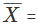1:75 m).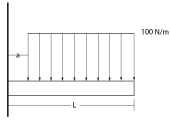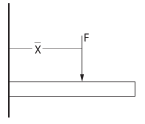Next, take the system shown below, a cantilevered beam with an increasing, triangular distributed load which peaks at w0.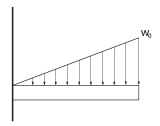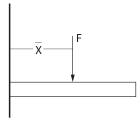The distributed load has units of force per unit length (N/m or lbs./ft.) and, in this case, can be written as,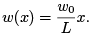(11.1)

In order to ensure that the forces in the two systems are equivalent, we require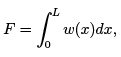(11.2)

which yields,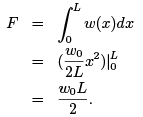We also want it to have the same moment about the origin. In order to do this we require that,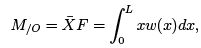(11.3)

which yields,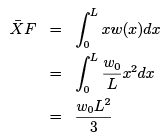and the position of the force,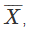must be 2/3 L

Example Here is another distributed load acting on a beam. Replace the distributed load with a single force.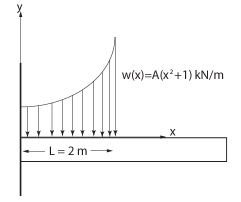Offer running on EduRev: Apply code STAYHOME200 to get INR 200 off on our premium plan EduRev Infinity!

## Engineering Mechanics

37 videos|85 docs|64 tests

,

,

,

,

,

,

,

,

,

,

,

,

,

,

,

,

,

,

,

,

,

;